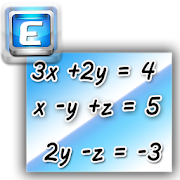# Linear Equation Solver

## Solving systems of linear / simultaneous equations.(Matrix or literally written)

3.58 EDUCATION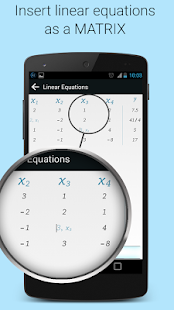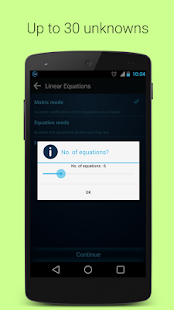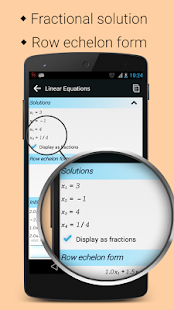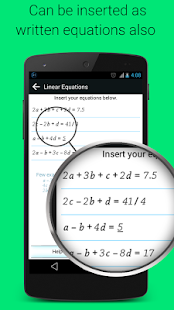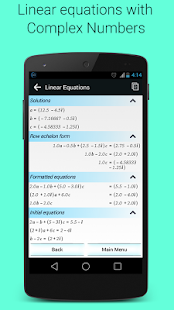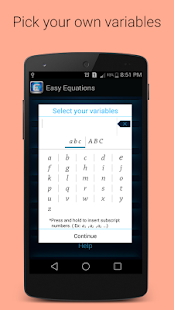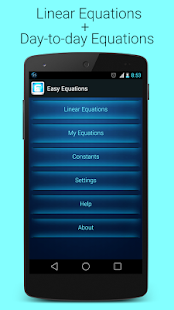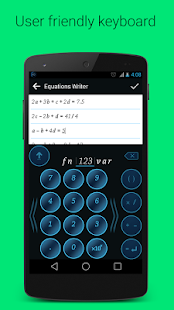"Easy Equations : Linear Equation Solver" is a smart application which solves a system of linear equations and general day-to-day equations easier than ever.

★ Ability to solve system of linear equations up to 30 unknowns.

Fractional or decimal solution.

★ Shows how the problem is being solved (Row echelon form).

★ Ability to solve linear equations with complex numbers.

★ Ability to use your own variables in linear equations (written equations mode). You can use subscripted numbers also. ( Ex: a1, a2, a3 ..)

★ Ability to solving systems of linear equations by inserting equations as the way they are written. Unlike in mostly available apps, you don’t need to format your equations. The app will do it for you.

★Ability to store any number of frequently used equations and constants as you prefer. (These could be non-linear or trigonometric as well.)

★ Allows you to make shortcuts for frequently used equations in your home screen. Few taps will get you the answer. (Awesome right?)

Works offline.

Welcome your new digital assistant in linear algebra. You will never regret of installing this once you start using the app!

NOTE: In mathematics, the theory of linear systems is the basis and a fundamental part of linear algebra, a subject which is used in most parts of modern mathematics. Computational algorithms for finding the solutions are an important part of numerical linear algebra, and play a prominent role in engineering, physics, chemistry, computer science, and economics. Linear equations with complex numbers is used in electrical engineering.

### Video

V 2.4 ★ The ad-free version can be activated by requesting the activation code for free ★ Minor UI changes and bug fixes V 2.3 ★ The solution can be displayed as fractions ★ Help documentation has been updated ★ Bug fixes and performance improvements ★ Improvements of the user interface

### Details and Recent Ratings

Size: 0 MB
Version: 2.6 by K. Dev
Updated: 15 October 2018 (1577 days ago)
Released: 24 March 2015
Installations: more than 100 000
5 Stars: 632
4 Stars: 92
3 Stars: 55
2 Stars: 148
1 Star: 260

### Similar apps

Android Game Categories
Android App Categories